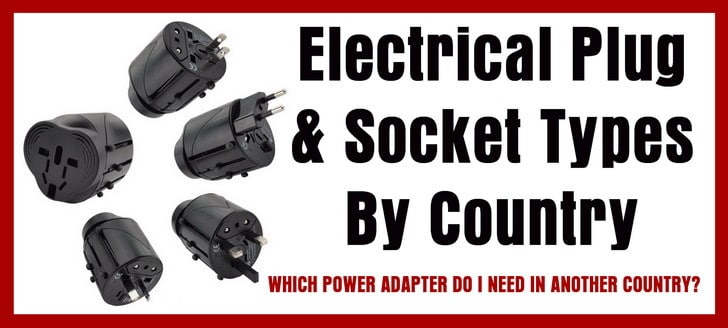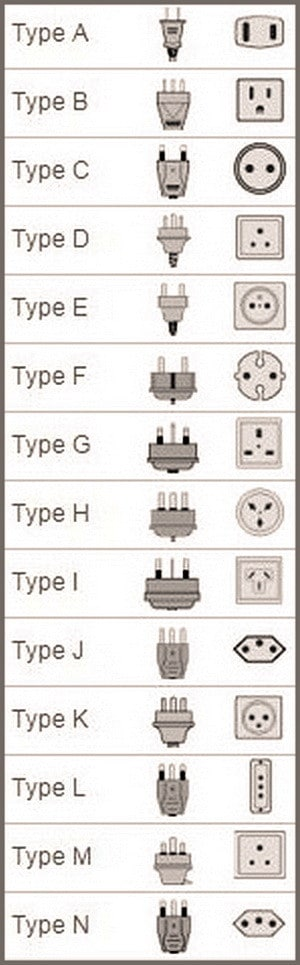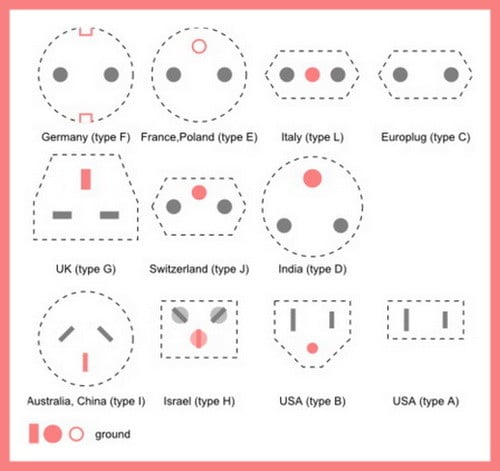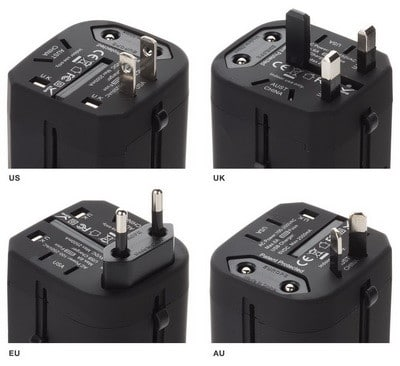# Electrical Plug and Socket Types By Country

Traveling and need to know the plug, outlet, and power voltage of that country? We list the most traveled countries types of power outlets, sockets, and plugs from around the world. The voltage, plug, and socket chart with descriptions will give you the information you need to understand what type of travel power adapter you will need to bring. Knowing what type of outlet is in the country you will be traveling to is absolutely essential. You will need to be able to charge your phone, tablet, laptop, or any other mobile devices while you are traveling.Plug and Socket Types By Country

Travel Hint: Bringing a universal travel power adapter with a compact power strip will solve all of your charging and power issues when traveling abroad. Universal travel power adapters have multiple plugs built into them for countries such as Australia, United Kingdom, New Zealand, Spain, France, Italy, EU, AU, UK, Germany, Mexico, Brazil, England, Ireland and more. A good universal travel power adapter will also have 1 or 2 USB connections/ports allowing you to charge your phone and tablet. You can then use the converted power outlet with a compact travel power strip allowing for many outlets to plug into.Electrical Plug and Socket Types By Country

### Argentina Voltage & Outlet Type:

The voltage is 220V and the frequency is 50Hz.
Argentina uses type C power sockets, and type I. (See chart above)

### Australia Voltage & Outlet Type:

The voltage is 230V and the frequency is 50Hz.
Australia uses type I power sockets. (See chart above)

### Austria Voltage & Outlet Type:

The voltage is 230V and the frequency is 50Hz.
Austria uses type F power sockets. (See chart above)

### Brazil Voltage & Outlet Type:

The voltage 127/220V and the frequency is 60Hz.
Brazilian uses type N power sockets. (See chart above)

### Cambodia Voltage & Outlet Type:

The standard voltage is 230V and the frequency is 50Hz.
Cambodia power sockets that are used are type A / C / G. (See chart above)

### Canada Voltage & Outlet Type:

The standard voltage is 120V and the frequency is 60Hz.
Canada power sockets used are type A and B. (See chart above)

### Caribbean Voltage & Outlet Type:

Aruba and Bahamas standard 120V and frequency is 60Hz.
Caribbean power sockets used are A / B / C / L. (See chart above)

### China Voltage & Outlet Type:

The standard voltage is 220V and the frequency is 50Hz.
China power sockets used are types A / C / I. (See chart above)

### Croatia Voltage & Outlet Type:

The standard voltage is 230V and the frequency is 50Hz.
Croatia power sockets are type F and work with plugs C and E. (See chart above)

### Denmark Voltage & Outlet Type:

The standard voltage is 230V and the frequency is 50Hz.
Denmark power sockets are type F and K. (See chart above)

### France Voltage & Outlet Type:

The standard voltage is 230V and the frequency is 50Hz.
French power sockets are type E. (See chart above)

### Germany Voltage & Outlet Type:

The standard voltage is 230V and the frequency is 50Hz.
German power sockets are type F. (See chart above)

### Greenland Voltage & Outlet Type:

The standard voltage is 230V and the frequency is 50Hz.
Greenland power sockets are type K. (See chart above)

### Hungary Voltage & Outlet Type:

The standard voltage is 230V and the frequency is 50Hz.
Hungary power sockets are type F. (See chart above)

### Iceland Voltage & Outlet Type:

The standard voltage is 230V and the frequency is 50Hz.
Iceland power sockets are type F. (See chart above)

### India Voltage & Outlet Type:

The standard voltage is 230V and the frequency is 50Hz.
Indian power sockets in use are types C / D / M. (See chart above)

### Indonesia Voltage & Outlet Type:

The standard voltage is 230V and the frequency is 50Hz.
Indonesian power sockets used are types C / F. (See chart above)

### Ireland Voltage & Outlet Type:

The standard voltage is 230V and the frequency is 50Hz.
Ireland power sockets used are type G. (See chart above)

### Italy Voltage & Outlet Type:

The standard voltage is 230V and the frequency is 50Hz.
Italian power sockets used are types F / L. (See chart above)

### Japan Voltage & Outlet Type:

The standard voltage is 100V and the frequency is 50/60Hz.
Japanese power sockets used are type A / B. (See chart above)

### South Korea Voltage & Outlet Type:

The standard voltage is 220V and the frequency is 60Hz.
South Korean power sockets used are types C / F. (See chart above)

### Malaysia Voltage & Outlet Type:

The standard voltage is 240V and the frequency is 50Hz.
Malaysian power sockets used are type G. (See chart above)

### Mexico Voltage & Outlet Type:

The standard voltage is 127V and the frequency is 60Hz.
Mexican power sockets used are types A / B. (See chart above)

### New Zealand Voltage & Outlet Type:

The standard voltage is 230V and the frequency is 50Hz.
New Zealand power sockets use type I. (See chart above)

### Peru Voltage & Outlet Type:

The standard voltage is 220V and the frequency is 60Hz.
Peru power sockets used are type A / B / C. (See chart above)

### Philippines Voltage & Outlet Type:

The standard voltage is 220V and the frequency is 60Hz.
Philippines power sockets use type A / B / C. (See chart above)

### Qatar Voltage & Outlet Type:

The standard voltage is 240V and the frequency is 50Hz.
Qatar power sockets used are types D / G. (See chart above)

### Russia Voltage & Outlet Type:

The standard voltage is 220V and the frequency is 50Hz.
Russian power sockets are types C / F. (See chart above)

### Spain Voltage & Outlet Type:

The standard voltage is 230V and the frequency is 50Hz.
Spanish power sockets used are type F. (See chart above)

### Sri Lanka Voltage & Outlet Type:

The standard voltage is 230V and the frequency is 50Hz.
Sri Lanka power sockets used are types D / G. (See chart above)

### Switzerland Voltage & Outlet Type:

The standard voltage is 230V and the frequency is 50Hz.
Swiss power sockets used are type J. (See chart above)

### Thailand Voltage & Outlet Type:

The standard voltage is 220V and the frequency is 50Hz.
Thailand power sockets used are types A / B / C. (See chart above)

### Turkey Voltage & Outlet Type:

The standard voltage is 230V and the frequency is 50Hz.
Turkish power sockets used are type F. (See chart above)

### United Arab Emirates Voltage & Outlet Type:

The standard voltage is 240V and the frequency is 50Hz.
UAE power sockets used are type G. (See chart above)

### United Kingdom Voltage & Outlet Type:

In the United Kingdom (England, Scotland, Ireland) the standard voltage is 230V and the frequency is 50Hz.
United Kingdom power sockets used are type G. (See chart above)

### Uruguay Voltage & Outlet Type:

The standard voltage is 220V and the frequency is 50Hz.
Uruguay power sockets that are used are type C / F / I / L. (See chart above)

### United States of America Voltage & Outlet Type:

The standard voltage is 120V and the frequency is 60Hz.
USA power sockets used are types A / B. (See chart above)

### Vietnam Voltage & Outlet Type:

The standard voltage is 220V and the frequency is 50Hz.
Vietnamese power sockets used are types A / C / G. (See chart above)Electrical Outlet Types By CountryUniversal power adapter for AU EU USA UK (with USB for mobile devices)

Are you traveling and need to know what type of outlet is in the country you are going to? Please leave a question below if you need information for a particular country and what plug, voltage, and outlets that country has.